4818.5 - Community Preparedness for Emergencies, Western Australia, October 2011
Latest ISSUE Released at 11:30 AM (CANBERRA TIME) 30/05/2012
Page tools:Print AllRSSSearch this Product

TECHNICAL NOTE DATA QUALITY

RELIABILITY OF ESTIMATES

1 Estimates in this publication are subject to non-sampling and sampling errors.

SAMPLING ERROR

2 As estimates in this publication are based on information obtained from a sample, they are subject to sampling variability. That is, they may differ from those estimates that would have been produced if all dwellings had been included in the survey.

3 One measure of the likely difference between the sample and population estimates is given by the standard error (SE), which indicates the extent to which an estimate might have varied by chance, because only a sample of dwellings (or occupants) was included.

4 There are about two chances in three (67%) that a sample estimate will differ by less than one SE from the number that would have been obtained if all dwellings had been included, and about 19 chances in 20 (95%) that the difference will be less than two SEs.

5 Another measure of the likely difference between the sample estimate and the population estimate is the relative standard error (RSE), which is obtained by expressing the SE as a percentage of the estimate:6 RSEs for estimates from the survey of Community Preparedness for Emergencies, WA, 2011 are published for each individual data cell. The Jackknife method of variance estimation is used for this process, which involves the calculation of 30 'replicate' estimates based on sub-samples of the original sample. The variability of estimates obtained from these sub-samples is used to estimate the sample variability surrounding the main estimate.

7 Limited publication space does not allow for the separate indication of the SEs and/or RSEs of all the estimates in this publication. However, RSEs for all these estimates are available free-of-charge on the ABS web site <www.abs.gov.au>.

8 In this publication, only estimates (numbers and proportions) with RSEs less than 25% are considered sufficiently reliable for most purposes. Estimates with RSEs between 25% and 50% have been included and are preceded by an asterisk (e.g. *3.4) to indicate they are subject to high uncertainty and should be used with caution.

9 Estimates with RSEs greater than 50% are preceded by a double asterisk (e.g. **2.1) to indicate that they are considered too unreliable for general use.

10 SEs can be calculated using the estimates (counts or proportions) and the corresponding RSEs. For example, Table 1 shows that the estimated number of households in Perth that have experienced any major emergencies was 41,100 (rounded to the nearest 100). The RSE table corresponding to the estimates in Table 1 (see Relative Standard Errors in the 'Relative Standard Error Table' section at the end of these Technical Notes) shows the RSE for this estimate is 14.6%. The SE is calculated by: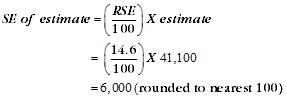11 Therefore, there are about two chances in three that the actual number of Perth households that have experienced any major emergencies was in the range of 35,100 to 47,100 and about 19 chances in 20 that the value was in the range of 29,100 to 53,100. This example is illustrated in the diagram below.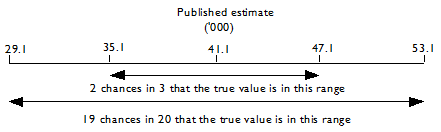ESTIMATION PROCEDURE

12 The estimates in this publication were obtained using a post-stratification procedure. This procedure ensured that the survey estimates conformed to an independently estimated distribution of the population, by state, part of state, age and sex rather than the observed distribution among respondents.

PROPORTIONS AND PERCENTAGES

13 Proportions and percentages formed from the ratio of two estimates are also subject to sampling errors. The size of the error depends on the accuracy of both the numerator and the denominator. A formula to approximate the RSE of a proportion is given below. This formula is only valid when the numerator (x) is a subset of the denominator (y).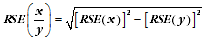14 As an example, using estimates from Table 1, of the approximately 665,900 households in Perth, 49.4%, that is approximately 329,100 households, will need to have their pets evacuated in a major emergency. The RSE for 329,100 is 2.5% and for 665,900 is 0.9% (see Relative Standard Errors in the 'Relative Standard Error Table' section at the end of these Technical Notes). Applying the above formula, the RSE for the proportion of households in Perth with pets needing evacuation in an emergency is: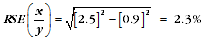15 Differences in the RSE calculated in the example above (2.3%) and the published RSE value (2.4%), are due to the use of rounded figures in the example above.

DIFFERENCES

16 Published estimates may also be used to calculate the difference between two survey estimates (of numbers or proportions). Such an estimate is also subject to sampling error. The sampling error of the difference between two estimates depends on their SEs and the relationship (correlation) between them. An approximate SE of the difference between two estimates (x-y) may be calculated by the following formula: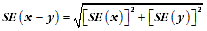17 While this formula will only be exact for differences between separate and uncorrelated characteristics or subpopulations, it provides a good approximation for all differences likely to be of interest in this publication.

SIGNIFICANCE TESTING

18 A statistical significance test for any comparisons between estimates can be performed to determine whether it is likely that there is a difference between two corresponding population characteristics. The standard error of the difference between two corresponding estimates (x and y) can be calculated using the formula in paragraph 16, above. The standard error is then used to create the following test statistic: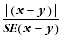19 If the value of this test statistic is greater than 1.96, then there is evidence, with a 95% level of confidence, of a statistically significant difference in the two populations with respect to that characteristic. Otherwise, it cannot be stated with confidence that there is a real difference between the populations with respect to that characteristic.

RELATIVE STANDARD ERROR TABLE

20 The RSE values for Table 1, used to calculate the figures in the examples described in paragraphs 10, 11 and 14, are given in the table below.

 Evacuation and selected household characteristics(a), by Perth Major Statistical Region(b) - WASTATISTICAL REGIONCentral Metropolitan Eastern Metropolitan Northern Metropolitan South West Metropolitan South East Metropolitan Perth MSR% % % % % % RSE of Households Experienced any major emergencies 47.2 31.9 30.7 34.8 24.5 14.6 Require assistance to exit dwelling 19.5 12.3 9.4 12.7 10.1 3.3 Require transport assistance 17.0 15.4 9.7 11.3 11.1 4.0 Have access to alternate accommodation 12.1 8.7 5.0 8.1 7.3 1.3 Pets needing evacuation 16.6 11.3 6.8 9.5 8.9 2.5 Provide unpaid care to non-household member 26.6 35.3 13.6 21.1 26.4 9.1 Unable to understand English np 35.3 20.3 np 25.3 12.5 Unable to speak English 59.5 37.1 21.5 38.0 24.4 11.8 Unable to understand emergency instructions in English np 59.8 31.0 np 30.5 np Not willing to evacuate 45.0 27.9 20.0 19.4 17.5 9.6 Total households 12.0 8.2 4.8 8.1 6.5 0.9 np not available for publication but included in totals where applicable, unless otherwise indicated (a) For details regarding Comparability of estimates over time, see Explanatory Notes 9 to 11. (b) Based on the 2010 edition of ASGC. See Explanatory Note 8 for details.

NON-SAMPLING ERROR

21 Non-sampling errors may arise as a result of errors in the reporting, recording or processing of data. These errors can be introduced through inadequacies in the questionnaire, treatment of non-response, inaccurate reporting by data providers, errors in the application of survey procedures, incorrect recording of answers and errors in data capture and processing.

22 The extent to which non-sampling error affects the results is difficult to measure. Every effort is made to minimise non-sampling error by careful design and testing of the collection instrument, intensive training and supervision of interviewers and the use of efficient operating procedures and systems.

COMPARABILITY OF ESTIMATES OVER TIME

23 In addition to the non-sampling errors outlined in the Reliability of statistics section, other factors may affect the comparability of estimates over with earlier iterations of this State Supplementary Survey topic. These factors are described in Explanatory notes 9 to 11.